# Lax-Milgram lemma

Many boundary-value problems for ordinary and partial differential equations can be posed in the following abstract variational form (cf. also Boundary value problem, ordinary differential equations; Boundary value problem, partial differential equations): Find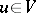such that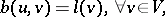(a1)

whereis a normed linear space (cf. also Norm),denotes a functional on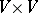andis an element in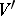(the dual of).

The essential question here is what conditions can be imposed on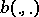and the normed spaceso that a unique solution to (a1) exists and depends continuously on the data.

The first result in this direction was obtained in 1954 by P.D. Lax and A.N. Milgram [a1], who established sufficient conditions for the existence and uniqueness of the solution for (a1).

Letbe a reflexive Banach space (cf. also Reflexive space) and let $b:V\times V\longrightarrow\mathbb{C}$ be a sesquilinear mapping (bilinear whenis real; cf. also Sesquilinear form) such that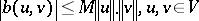(continuity) and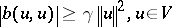(strong coercivity), where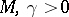. Then there exists a unique bijective linear mapping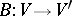, continuous in both directions and uniquely determined by, with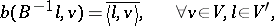and for the norms one has: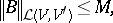This implies thatis the solution of (a1). The above theorem only establishes existence of a solution to (a1), namely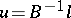, but does not say anything about the construction of this solution. In 1965, W.V. Petryshyn [a2] proved the following result: Letbe a separable reflexive Banach space (cf. also Separable space),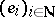a basis ofanda continuous sesquilinear strongly coercive mapping on. Then for all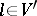:

i) for all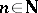the systemis uniquely solvable for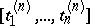;

ii) the sequence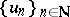determined by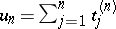converges into athat is the solution of (a1).

To see that the strong coerciveness property of the sesquilinear mappingis not necessary for the existence of the solution to (a1), consider the following very simple example.

Let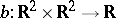be defined by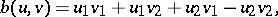where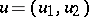,. It is easy to see thatis bilinear and continuous. It is not strongly coercive, because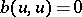when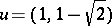. However, for all,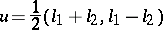is the unique solution to (a1).

In 1971, I. Babuška [a3] gave a significant generalization of the Lax–Milgram theorem using weak coerciveness (cf. Babuška–Lax–Milgram theorem).

An extensive literature exists on applications of the Lax–Milgram lemma to various classes of boundary-value problems (see, e.g., [a4], [a5]).

How to Cite This Entry:
Lax-Milgram lemma. Encyclopedia of Mathematics. URL: http://encyclopediaofmath.org/index.php?title=Lax-Milgram_lemma&oldid=27194
This article was adapted from an original article by I. RoÅŸca (originator), which appeared in Encyclopedia of Mathematics - ISBN 1402006098. See original article